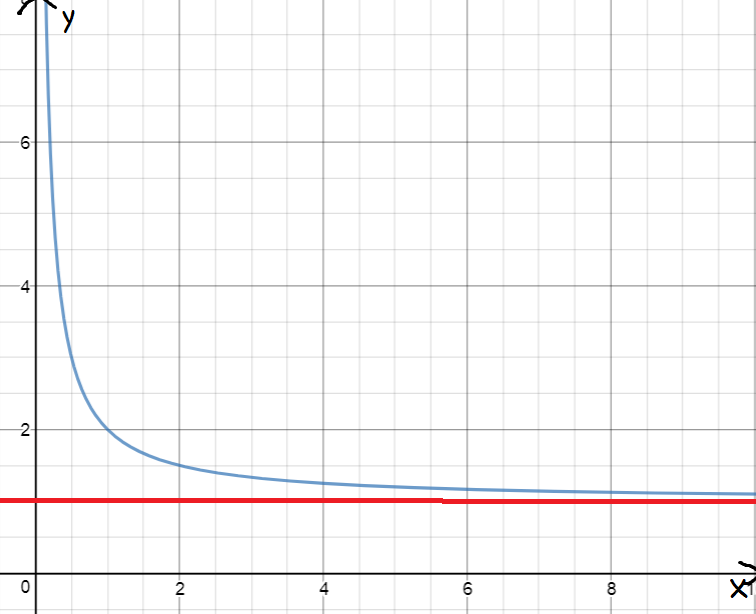Definition of LimitA limit is a value that a function (or sequence) gets closer and closer to, but may not quite reach. It is a value that the function approaches.

For example, the picture shows the graph of $y = 1 + \dfrac{1}{x}$. We can see that the graph gets closer and closer to the line $y = 1$, but it never quite gets there.

We say that $1 + \dfrac{1}{x}$ approaches $1$ as $x$ approaches infinity (gets larger and larger). We use the following notation to write this down:

$\displaystyle{\lim_{x \to \infty} \left( 1 + \dfrac{1}{x}\right) = 1 }.$

Description

The aim of this dictionary is to provide definitions to common mathematical terms. Students learn a new math skill every week at school, sometimes just before they start a new skill, if they want to look at what a specific term means, this is where this dictionary will become handy and a go-to guide for a student

Audience

Year 1 to Year 12 students

Learning Objectives

Learn common math terms starting with letter L

Author: Subject Coach